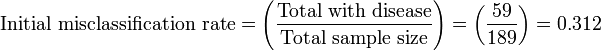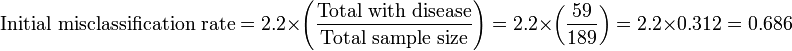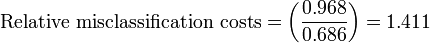# Recursive partitioning

## Contents

In statistics, recursive partitioning is a method for the multivariable analysis of diagnostic tests.. Recursive partitioning creates a decision tree that strives to correctly classify members of the population based on a dichotomous dependent variable. Compared to other multivariable:

• Generates clinically more intuitive models that do not require the user to perform calculations.
• Allows "adjustable misclassification penalties" or misclassification costs in order to create a decision rule that has more sensitivity or specificity. This has also been called the "diversity index" which is the sum of the false-negatives and false-positives. Either the false-negatives and false-positives can be weighted in order to preferentially reduce their occurrence.
• Does not work well for continuous variables
• May overfit data.

Studies comparing the predictive accuracy of recursive partitioning with logistic regression have reported no difference, recursive partitioning to be more accurate and logistic regression to be more accurate.

A variation is 'Cox linear recursive partitioning'.

Examples are available of using recursive partitioning in research of diagnostic tests. Goldman used recursive partitioning to prioritize sensitivity in the diagnosis of myocardial infarction among patients with chest pain in the emergency room.

Additional alternatives to recursive partitioning include latent class analysis.

## Calculations

### Unweighted analysis

An 'Initial misclassification rate' is calculated by assigning all cases to a single class. Using the example in the CART Manual, in trying to diagnose 59 patients who have a disease of interest among a sample of 189 patients, arbitrarily classifying all patients as normal yields:
For the top node, also called node 1:$\mbox{Initial misclassification rate} =\left (\frac{\mbox{Total with disease}}{\mbox{Total sample size}}\right)=\left (\frac{\mbox{59}}{\mbox{189}}\right)=\mbox{0.312}$

For the next nodes: The next best independent, classifier variable creates two nodes:

• Left node: 159 patients; 118 have disease and 41 are false positives who do not have the disease
• Right node: 30 patients; 12 have disease and false negatives$\mbox{Misclassification costs} =\left (\frac{\mbox{41}}{\mbox{159}}\right) + \left (\frac{\mbox{12}}{\mbox{30}}\right)=\left (\mbox{0.258}\right) + \left(\mbox{0.400}\right)=\mbox{0.658}$$\mbox{Relative misclassification costs} =\left (\frac{\mbox{0.658}}{\mbox{0.312}}\right)=\mbox{2.109}$

### Weighted analysis

In this analysis, the sensitivity is preferenced by weighted it by 2.2, which is the ratio of patients without disease over patients with disease.
For the top node:$\mbox{Initial misclassification rate} =2.2 \times \left (\frac{\mbox{Total with disease}}{\mbox{Total sample size}}\right)=2.2 \times \left (\frac{59}{189}\right)=2.2 \times 0.312 = 0.686$

For the next nodes: The next best independent, classifier variable creates two nodes:

• Left node: 159 patients; 118 have disease and 41 are false positives who do not have the disease
• Right node: 30 patients; 12 have disease and false negatives$\mbox{Misclassification costs} =2.2 \times \left (\frac{41}{159}\right) + \left (\frac{12}{30}\right)=2.2 \times 0.258 + 0.400 = 0.568 + 0.400 = 0.968$$\mbox{Relative misclassification costs} =\left (\frac{0.968}{0.686}\right)= 1.411$

### Stopping rules

Various methods have been proposed to avoid overfitting of data.

• One method is to grow the tree as far as possible, then to 'prune' the tree. Pruning is based on a balance between misclassification costs and tree complexity.

Another method is to grow the tree "until p<0.05 or until one or more of the marginal counts in the two-by-two table is less than 10."

## Software for recursive partitioning

Software available for recursive partitioning includes: